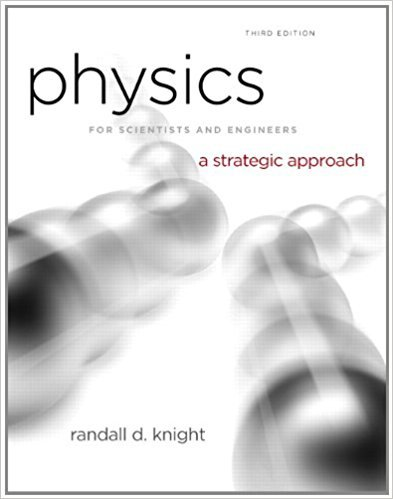×
Log in to StudySoup
Get Full Access to Physics For Scientists And Engineers: A Strategic Approach With Modern Physics - 3 Edition - Chapter 1 - Problem 54p
Join StudySoup for FREE
Get Full Access to Physics For Scientists And Engineers: A Strategic Approach With Modern Physics - 3 Edition - Chapter 1 - Problem 54p

Already have an account? Login here
×
Reset your password

# The quantity called mass density is the mass per unitISBN: 9780321740908 69

## Solution for problem 54P Chapter 1

Physics for Scientists and Engineers: A Strategic Approach with Modern Physics | 3rd Edition

• Textbook Solutions
• 2901 Step-by-step solutions solved by professors and subject experts
• Get 24/7 help from StudySoup virtual teaching assistantsPhysics for Scientists and Engineers: A Strategic Approach with Modern Physics | 3rd Edition

4 5 1 243 Reviews
14
5
Problem 54P

Problem 54P

The quantity called mass density is the mass per unit volume of a substance. Express the following mass densities in SI units.

a. Aluminum, 2.7 × 10−3 kg/cm3

b. Alcohol, 0.81 g/cm3

Step-by-Step Solution:

Solution 54P

Part (a)

Step 1 of 2:

We are going to convert the given mass density in SI units.

The mass density of AluminumThe mass density of Aluminum is 2.7 × 103 kg/m3.

Part (b)

Step 2 of 2

##### ISBN: 9780321740908

The full step-by-step solution to problem: 54P from chapter: 1 was answered by , our top Physics solution expert on 08/30/17, 04:34AM. The answer to “The quantity called mass density is the mass per unit volume of a substance. Express the following mass densities in SI units.a. Aluminum, 2.7 × 10?3 kg/cm3b. Alcohol, 0.81 g/cm3” is broken down into a number of easy to follow steps, and 30 words. This textbook survival guide was created for the textbook: Physics for Scientists and Engineers: A Strategic Approach with Modern Physics, edition: 3. Physics for Scientists and Engineers: A Strategic Approach with Modern Physics was written by and is associated to the ISBN: 9780321740908. This full solution covers the following key subjects: mass, alcohol, called, densities, Density. This expansive textbook survival guide covers 17 chapters, and 1439 solutions. Since the solution to 54P from 1 chapter was answered, more than 497 students have viewed the full step-by-step answer.

Unlock Textbook Solution

Enter your email below to unlock your verified solution to:

The quantity called mass density is the mass per unit